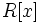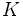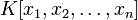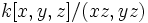# Cohen-Macaulay ring

(Redirected from CM ring)
Jump to: navigation, search
This article is about a definition in group theory that is standard among the commutative algebra community (or sub-community that dabbles in such things) but is not very basic or common for people outside

View a list of other standard non-basic definitions
This article defines a property of commutative unital rings; a property that can be evaluated for a commutative unital ring
View all properties of commutative unital rings
VIEW RELATED: Commutative unital ring property implications | Commutative unital ring property non-implications |Commutative unital ring metaproperty satisfactions | Commutative unital ring metaproperty dissatisfactions | Commutative unital ring property satisfactions | Commutative unital ring property dissatisfactions

## Definition

### Symbol-free definition

A commutative unital ring is termed Cohen-Macaulay if it is Noetherian and satisfies the following equivalent conditions:

### Equivalence of definitions

Further information: Equivalence of definitions of Cohen-Macaulay

## Metaproperties

Metaproperty name Satisfied? Proof Statement with symbols
polynomial-closed property of commutative unital rings Yes Cohen-Macaulay is polynomial-closed Suppose$R$ is a Cohen-Macaulay ring. Then, the polynomial ring$R[x]$ is also a Cohen-Macaulay ring.
strongly local property of commutative unital rings Yes Cohen-Macaulay is strongly local The following are eqiuvalent for a commutative unital ring$R$: (i)$R$ is Cohen-Macaulay, (ii) the localization at any prime ideal of$R$ is Cohen-Macaulay, (iii) the localization at any maximal ideal of$R$ is Cohen-Macaulay.
quotient-closed property of commutative unital rings No Cohen-Macaulay is not quotient-closed It is possible to have a Cohen-Macaulay ring$R$ and an ideal$I$ in$R$ such that$R/I$ is not Cohen-Macaulay. However, if$I$ is generated by a regular sequence, then the quotient is a Cohen-Macaulay ring. Thus, for instance, the quotient by a principal ideal generated by an element which is not a zero divisor is again a Cohen-Macaulay ring.
finite direct product-closed property of commutative unital rings Yes Cohen-Macaulay is finite direct product-closed Suppose$R_1,R_2,\dots,R_n$ are all Cohen-Macaulay rings. Then, the external direct product$R_1 \times R_2 \times \dots \times R_n$ is also a Cohen-Macaulay ring.

## Relation with other properties

### Conjunction with other properties

Conjunction Other component of conjunction Comments
Cohen-Macaulay domain integral domain
local Cohen-Macaulay ring local ring

### Stronger properties

Property Meaning Proof of implication Proof of strictness (reverse implication failure) Intermediate notions
Regular local ring regular local implies Cohen-Macaulay Cohen-Macaulay not implies regular local click here
regular ring its localization at any prime ideal is a regular local ring Regular implies Cohen-Macaulay Cohen-Macaulay not implies regular click here
polynomial ring over a field has the form$K[x]$ where$K$ is a field click here
multivariate polynomial ring over a field has the form$K[x_1,x_2,\dots,x_n]$ where$K$ is a field click here
principal ideal domain every ideal is a principal ideal, i.e., it is generated by one element click here
Dedekind domain one-dimensional Noetherian normal domain click here
Finite-dimensional algebra over a field click here
Artinian ring every descending chain of ideals stabilizes at a finite length Artinian implies Cohen-Macaulay click here
one-dimensional Noetherian domain one-dimensional Noetherian integral domain One-dimensional Noetherian domain implies Cohen-Macaulay click here

### Weaker properties

Property Meaning Proof of implication Proof of strictness (reverse implication failure) Intermediate notions
Noetherian ring every ideal is finitely generated click here
universally catenary ring Cohen-Macaulay implies universally catenary universally catenary not implies Cohen-Macaulay click here
catenary ring Cohen-Macaulay implies catenary catenary not implies Cohen-Macaulay click here

## Spectrum

The spectrum of a Cohen-Macaulay ring has the following important geometric property: If two irreducible components intersect, they must have the same dimension. Here, by the dimension of an irreducible component, we mean the dimension of the corresponding minimal prime ideal, or more explicitly, the Krull dimension of the quotient ring by that minimal prime ideal.

This rules out, for instance, rings like$k[x,y,z]/(xz,yz)$.

However:

• The spectrum need not be irreducible: The ring$k[x,y]/(xy)$ is Cohen-Macaulay, although its spectrum has two irreducible components.
• All components of the spectrum need not have the same dimension: For instance, we could have a ring that is a disjoint union of irreducible subsets of different dimensions
• Not every irreducible space is the spectrum of a Cohen-Macaulay ring. In other words, not every integral domain, or even every affine domain, is Cohen-Macaulay,